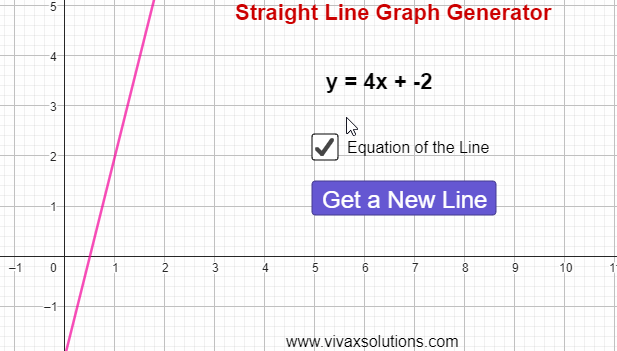### Straight Line Graph Generator: for Year 9, GCSE MathsThe equation of a straight line is given by the formula, y = mx + c, where m and c are the gradient and intercept respectively.

The gradient, m, is the slope or steepness of the line; the intercept, c, is the point where it crosses the y-axis at.

E.g.
A straight line passes through (1,2) and (3, 8). Find its equation.
m = (8-2)/(3-1) = 3
m = 3 x = 1 y = 2
y = mx + c
2 = 3(1) + c
c =  -1
The equation of the straight line is y = 3x - 1.

Using the following simulation, you can create lines at random, calculate the equation as above and then check the answer, in order to be highly competent in the topic.